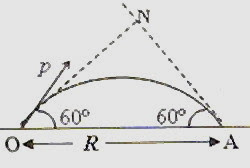## Saturday, February 28, 2009

### Angular Momentum- Two Multiple Choice Questions

How strange is the lot of us mortals! Each of us is here for a brief sojourn; for what purpose we know not, though some times sense it. But we know from daily life that we exist for other people first of all for whose smiles and well-being our happiness depends.

– Albert Einstein

(1) A thin uniform wooden rod AB of length L and mass M is hinged without friction at the end B. A small block of clay of mass m moving horizontally with velocity v srikes the end B of the rod and gets stuck to it. The angular velocity of the system about A just after the collision is

(b) 3mv/(mL + ML)

(c) (mv+ ML)/ 3mL

(d) mv/(3mL + ML)

(e) 3mv/(3mL + ML)

The angular momentum of the system about the point A just before collision is the angular momentum of the block of clay which is equal to mvL. (Note that the perpendicular distance of the line of action of the linear momentum (mv) of the block of clay from the point A is L).

The angular momentum of the system about the point A just after the collision is where I is the total moment of inertia of the rod and clay and ω is the angular velocity of the system immediately after the collision.

We have I = ML2/3 + mL2

Equating the angular momenta before and after collision, we have

mvL = (ML2/3 + mL2) ω

Therefore ω = 3mv/(3mL + ML).

(2) A particle is projected at an angle of 60º with the horizontal with linear momentum of magnitude p. The horizontal range of this projectile is R. Just before the projectile strikes the ground at A, what is the magnitude of its angular momentum aboutan axis perpendicular the plane of motion and passing through the point of projection? Neglect air resistance.

(a) pR

(b) pR/2

(c) (√3) pR /2

(d) (√3) pR

(e) Zero

This is a very simple question. The magnitude of the linear momentum of the projectile just before it strikes the ground will be equal to p. The magnitude of the angular momentum at the moment will be p×Lever arm = p×ON = p×R sin 60º = (√3) pR /2.

#### 1 comment:

1.Anonymous11:37 AM

This is very attention-grabbing, You're an overly professional blogger.
I've joined your rss feed and stay up for in quest of more of your fantastic post.
Also, I've shared your web site in my social networks

Here is my web page :: pandora profits